Hostname: page-component-546b4f848f-fhndm Total loading time: 0 Render date: 2023-06-04T19:51:37.175Z Has data issue: false Feature Flags: { "useRatesEcommerce": true } hasContentIssue false

# Quotients of A2 * T2

Published online by Cambridge University Press:  20 November 2018

## Abstract

HTML view is not available for this content. However, as you have access to this content, a full PDF is available via the ‘Save PDF’ action button.

We study unitary quotients of the free product unitary pivotal category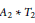${{A}_{2}}\,*\,{{T}_{2}}$. We show that such quotients are parametrized by an integer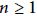$n\,\ge \,1$ and an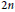$2n$–th root of unity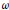$\omega$. We show that for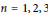$n\,=\,1,\,2,\,3$, there is exactly one quotient and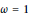$\omega \,=\,1$. For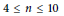$4\,\le \,n\,\le \,10$, we show that there are no such quotients. Our methods also apply to quotients of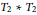${{T}_{2}}\,*\,{{T}_{2}}$, where we have a similar result.

The essence of our method is a consistency check on jellyfish relations. While we only treat the specific cases of${{A}_{2}}\,*\,{{T}_{2}}$ and${{T}_{2}}\,*\,{{T}_{2}}$, we anticipate that our technique can be extended to a general method for proving the nonexistence of planar algebras with a specified principal graph.

During the preparation of this manuscript, we learnt of Liu's independent result on composites of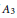${{A}_{3}}$ and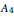${{A}_{4}}$ subfactor planar algebras (arxiv:1308.5691). In 1994, Bisch–Haagerup showed that the principal graph of a composite of${{A}_{3}}$ and${{A}_{4}}$ must fit into a certain family, and Liu has classified all such subfactor planar algebras. We explain the connection between the quotient categories and the corresponding composite subfactor planar algebras. As a corollary of Liu's result, there are no such quotient categories for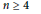$n\,\ge \,4$.

This is an abridged version of arxiv:1308.5723.

## Keywords

Type
Research Article
Information
Canadian Journal of Mathematics , 01 October 2016 , pp. 999 - 1022

## References

[BHP12] Brothier, A., Hartglass, M., and Penneys, D., Rigid C*-tensor categories ofbimodules over interpolated free group factors. J. Math. Phys. 53(2012), no. 12,123525. http://dx.doi.Org/10.1 063/1.4769178 CrossRefGoogle Scholar
[BiglO] Bigelow, S., Skein theory for the ADE planar algebras. J. Pure Appl. Algebra 214(2010), no.5, 658666. http://dx.doi.Org/10.1 01 6/j.jpaa.2009.07.010 Google Scholar
[Bis94] Bisch, D., A note on intermediate subfactors. Pacific J. Math. 163(1994), no. 2, 201216. http://dx.doi.org/10.2140/pjm.1994.163.201 CrossRefGoogle Scholar
[BJ97] Bisch, D., Algebras associated to intermediate subfactors. Invent. Math. 128(1997), 89157. http://dx.doi.Org/10.1 OO7/sOO222OO5O137 Google Scholar
[BL10] Bhattacharyya, B. and Landau, Z., Intermediate standard invariants and intermediate planar algebras. 2010. http://www.eecs.berkeley.edu/∼landau/publications.htm Google Scholar
[BMPS12] Bigelow, S., Morrison, S., Peters, E., and Snyder, N., Constructing the extended Haagerup planar algebra. Acta Math. 209(2012), no. 1, 2982. http://dx.doi.Org/10.1007/s11 511 -012-0081 -7 CrossRefGoogle Scholar
[Gol59] Goldman, M., On subfactors of factors of type II1. Michigan Math. J. 6(1959), 167172. http://dx.doi.Org/10.1307/mmj71028998188 Google Scholar
[GS12] Grossman, P. and Snyder, N., Quantum subgroups of the Haagerup fusion categories. Comm.Math. Phys. 311(2012), no. 3, 617643. http://dx.doi.Org/10.1OO7/sOO22O-O12-1427-x CrossRefGoogle Scholar
[Haa94] Haagerup, U., Principal graphs of subfactors in the index range 4 < [M < : N] < 3 + \2. In: Subfactors (Kyuzeso, 1993), World Sci. Publ., River Edge, NJ, 1994, pp. 138.Google Scholar
[IK93] Izumi, M. and Kawahigashi, Y., Classification of subfactors with the principal graph D(1) n. J.Funct. Anal. 112(1993), no. 2, 257286. http://dx.doi.org/10.1006/jfan.1993.1033 CrossRefGoogle Scholar
[IMP13] Izumi, M., Morrison, S., and Penneys, D., Quotients of A2 * T2. Canad. J. Math., to appear;extended version available as Fusion categories between C ⊠ D and C * D. arxiv:1308.5723.Google Scholar
[LanO2] Landau, Z. A., Exchange relation planar algebras. In: Proceedings of the Conference on Geometric and Combinatorial Group Theory, Part II (Haifa, 2000), Geom. Dedicata 95(2002), 183214. http://dx.doi.Org/10.1023/A:1021296230310 Google Scholar
[Liul3] Liu, Z., Composed inclusions of A3 and A4 subfactors. Adv. Math. 279(2015), 307371. http://dx.doi.Org/10.1016/j.aim.2O15.03.017Google Scholar
[MP14] Morrison, S. and Penneys, D., 2-supertransitive subfactors at index3 + \5.J. Funct. Anal. 269(2015), no. 9, 28452870. http://dx.doi.Org/10.1016/j.jfa.2015.06.023 CrossRefGoogle Scholar
[MPPS12] Morrison, S., Penneys, D., E.|Peters, and Snyder, N. , Subfactors of index less than 5, Part 2: Triple points. Internat. J. Math. 23(2012), 1250016, 33. http://dx.doi.Org/10.1142/S0129167X11007586 CrossRefGoogle Scholar
[Pop94] Popa, S., Classification of amenable subfactors of type II. Acta Math. 172(1994), no. 2, 163255. http://dx.doi.org/10.1007/BF02392646 CrossRefGoogle Scholar
[PP13] Penneys, D. and Peters, E., Calculating two-strand jellyfish relations. Pacific J. Math. 277(2015), no. 2, 463510. http://dx.doi.org/10.2140/pjm.2015.277.463 CrossRefGoogle Scholar